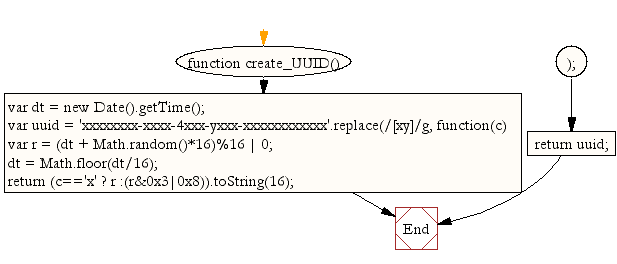# JavaScript: Function to create a UUID identifier

## JavaScript Math: Exercise-23 with Solution

Write a JavaScript function to create a UUID identifier.

Note :
According to Wikipedia - A universally unique identifier (UUID) is an identifier standard used in software construction. A UUID is simply a 128-bit value. The meaning of each bit is defined by any of several variants. For human-readable display, many systems use a canonical format using hexadecimal text with inserted hyphen characters. For example : de305d54-75b4-431b-adb2-eb6b9e546014

Sample Solution:-

HTML Code:

``````<!DOCTYPE html>
<html>
<meta charset="utf-8">
<title>Write a JavaScript function to create a UUID</title>
<body>

</body>
</html>
```
```

JavaScript Code:

``````function create_UUID(){
var dt = new Date().getTime();
var uuid = 'xxxxxxxx-xxxx-4xxx-yxxx-xxxxxxxxxxxx'.replace(/[xy]/g, function(c) {
var r = (dt + Math.random()*16)%16 | 0;
dt = Math.floor(dt/16);
return (c=='x' ? r :(r&0x3|0x8)).toString(16);
});
return uuid;
}

console.log(create_UUID());
```
```

Sample Output:

```1fe35579-5ce7-46ec-89e0-7e7236700297
```

Flowchart:Live Demo:

See the Pen javascript-math-exercise-23 by w3resource (@w3resource) on CodePen.

Improve this sample solution and post your code through Disqus

What is the difficulty level of this exercise?

Test your Programming skills with w3resource's quiz.

﻿

## JavaScript: Tips of the Day

Reduce method

```[1, 2, 3, 4].reduce((x, y) => console.log(x, y));
```

The first argument that the reduce method receives is the accumulator, x in this case. The second argument is the current value, y. With the reduce method, we execute a callback function on every element in the array, which could ultimately result in one single value.
In this example, we are not returning any values, we are simply logging the values of the accumulator and the current value.
The value of the accumulator is equal to the previously returned value of the callback function. If you don't pass the optional initialValue argument to the reduce method, the accumulator is equal to the first element on the first call.
On the first call, the accumulator (x) is 1, and the current value (y) is 2. We don't return from the callback function, we log the accumulator and current value: 1 and 2 get logged.
If you don't return a value from a function, it returns undefined. On the next call, the accumulator is undefined, and the current value is 3. undefined and 3 get logged.
On the fourth call, we again don't return from the callback function. The accumulator is again undefined, and the current value is 4. undefined and 4 get logged.

Ref: https://bit.ly/323Y0P6

We are closing our Disqus commenting system for some maintenanace issues. You may write to us at reach[at]yahoo[dot]com or visit us at Facebook